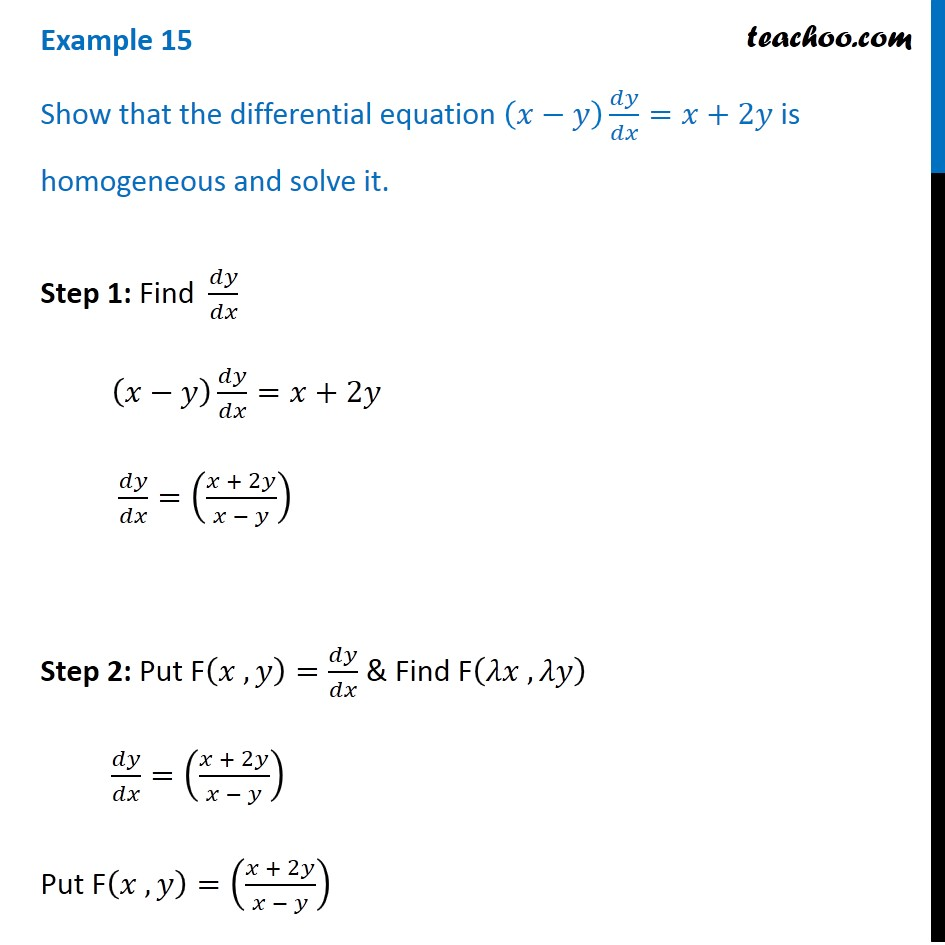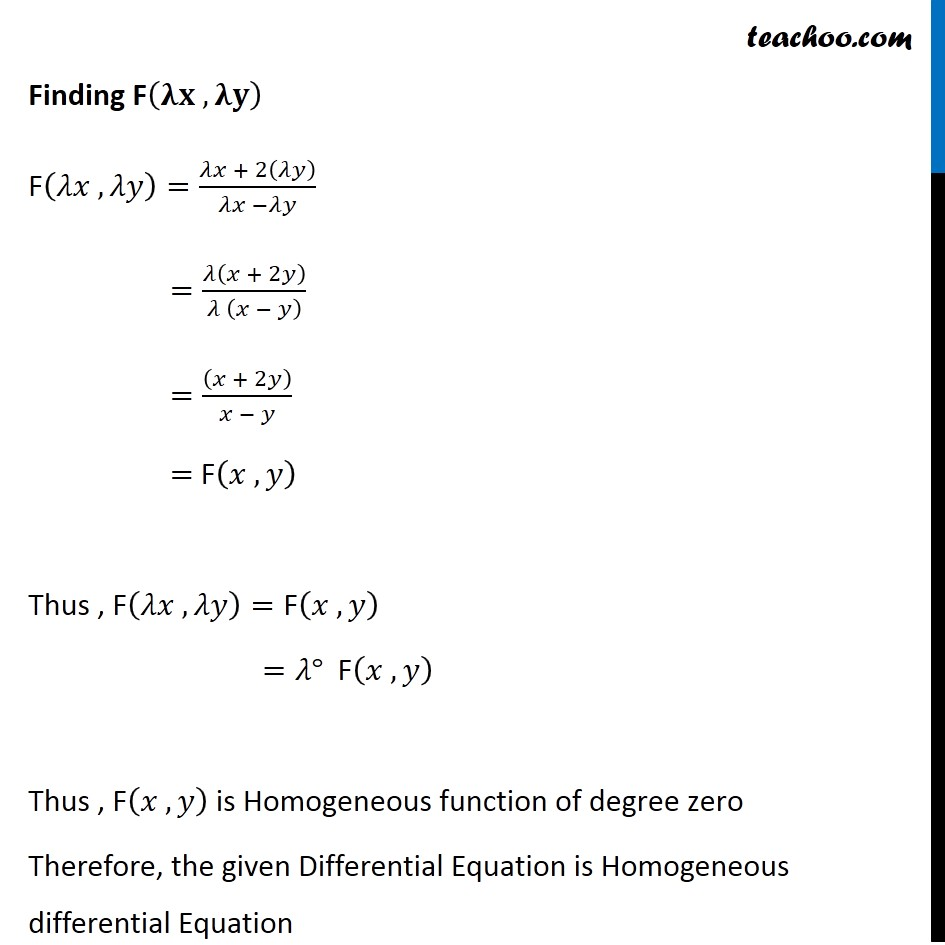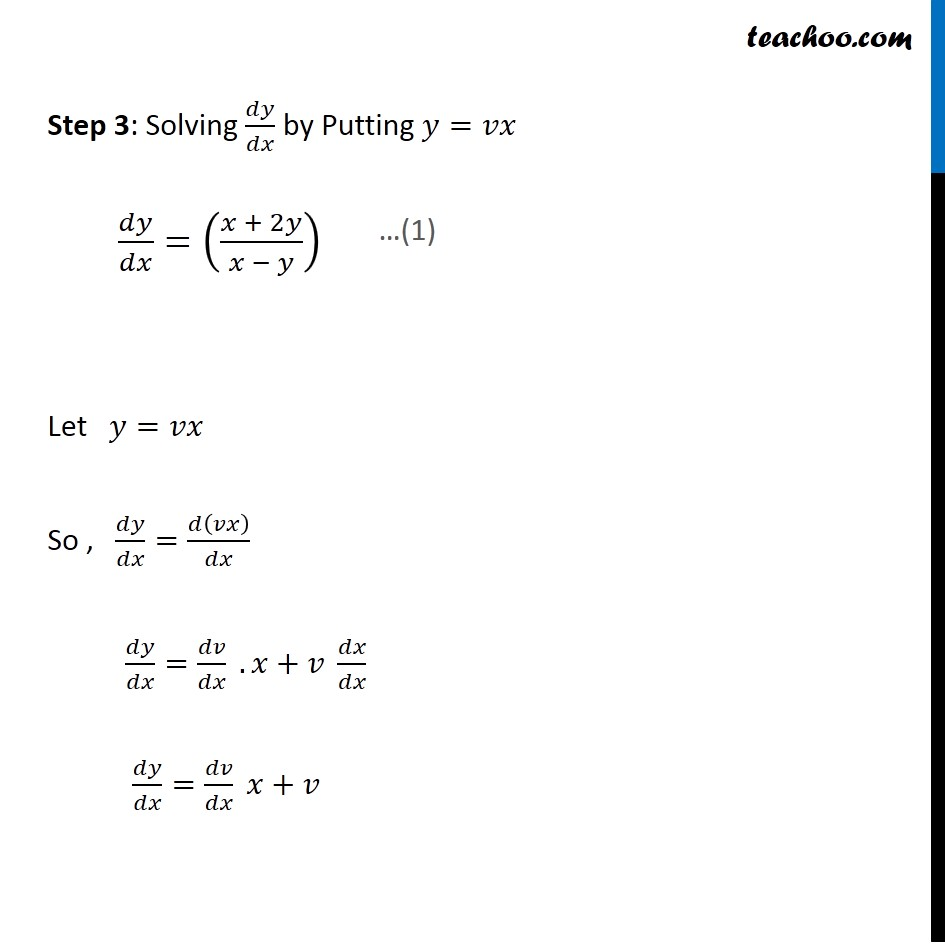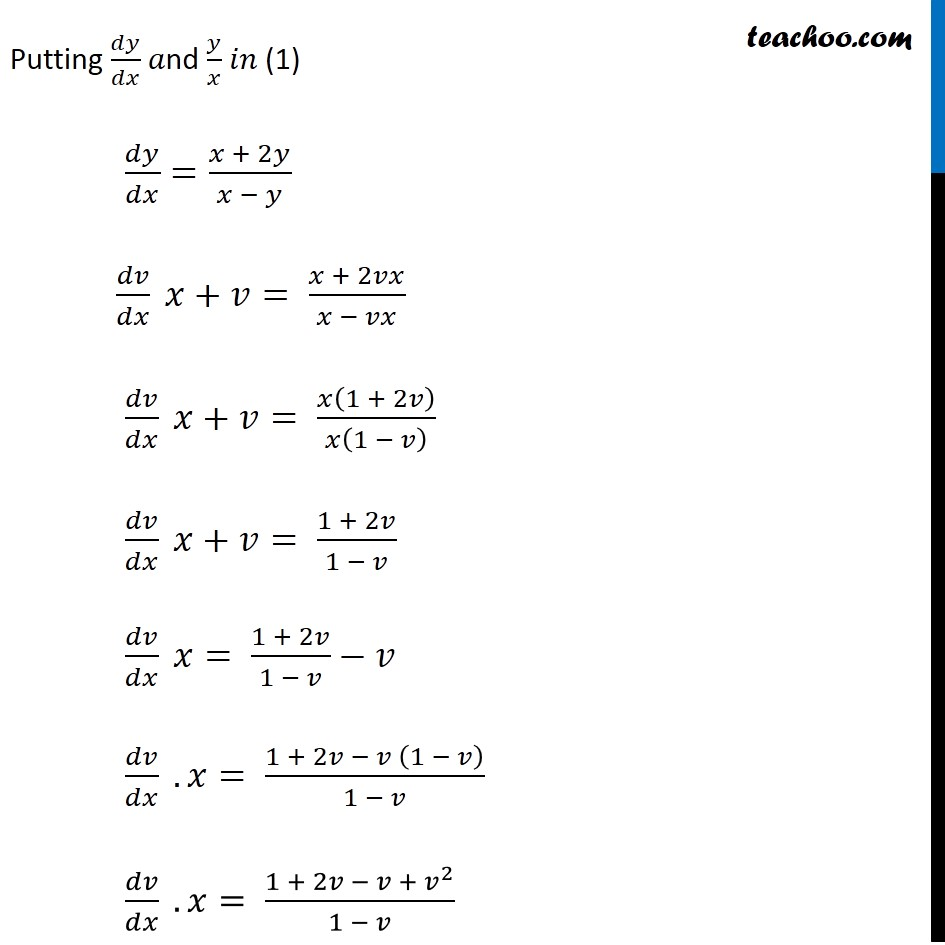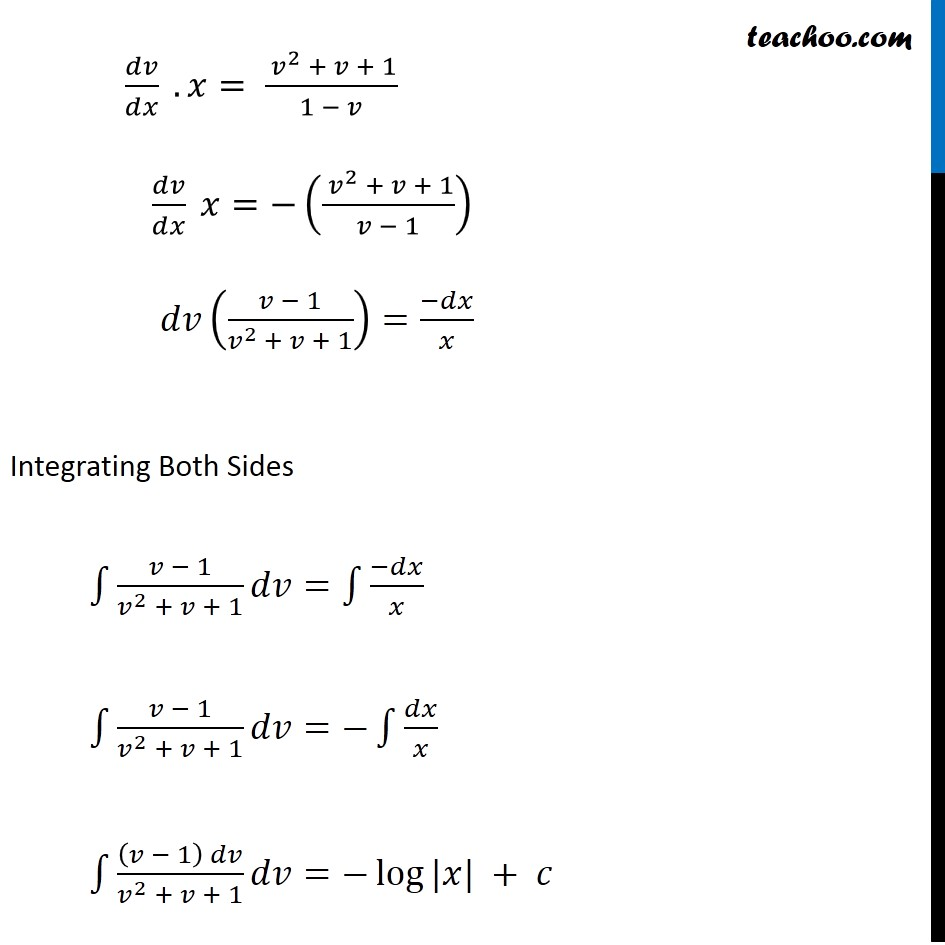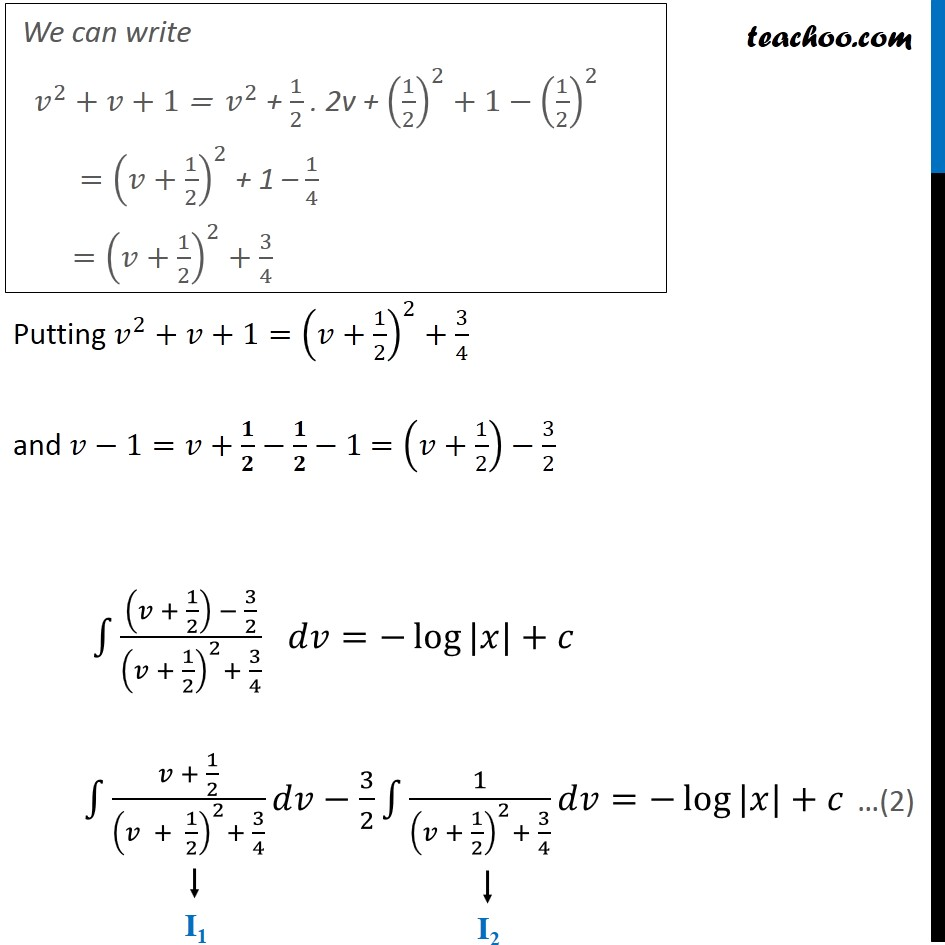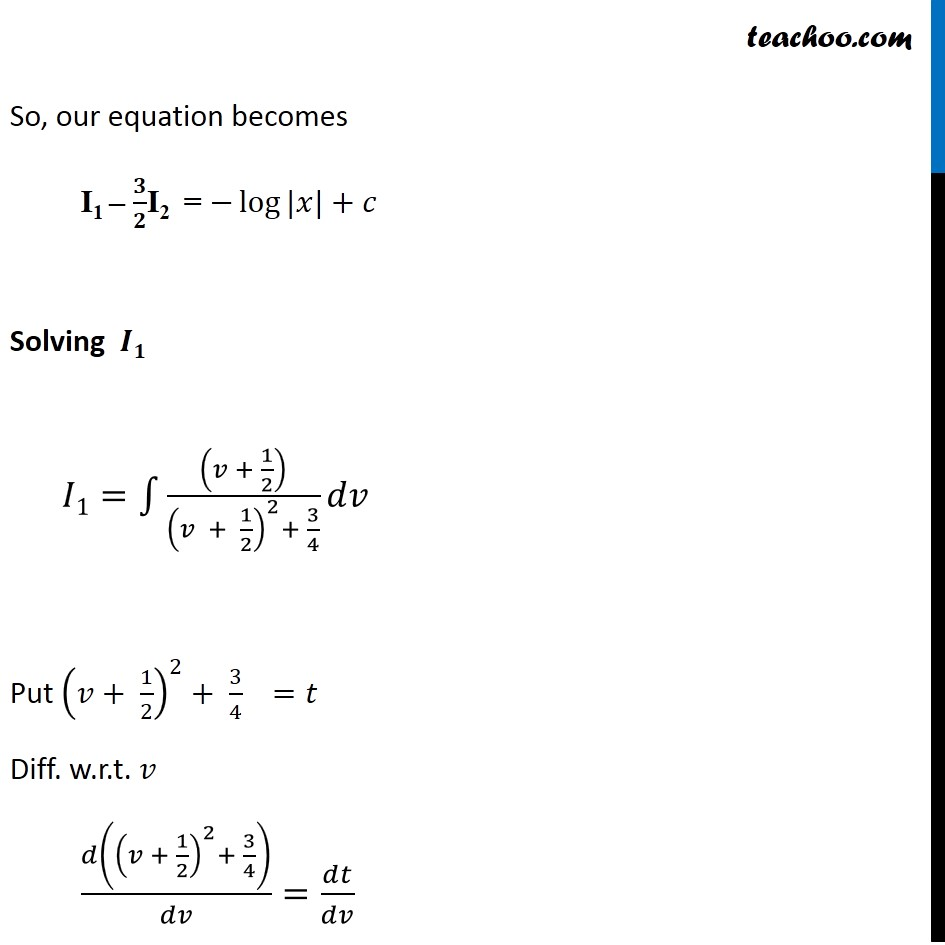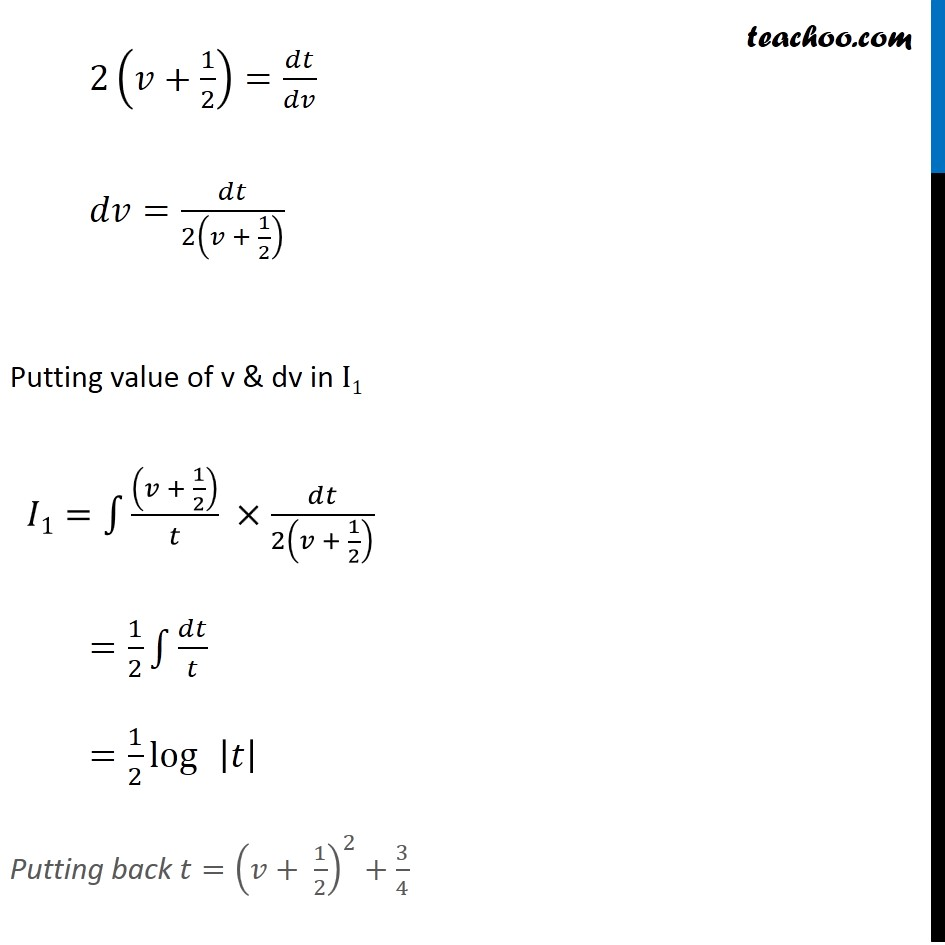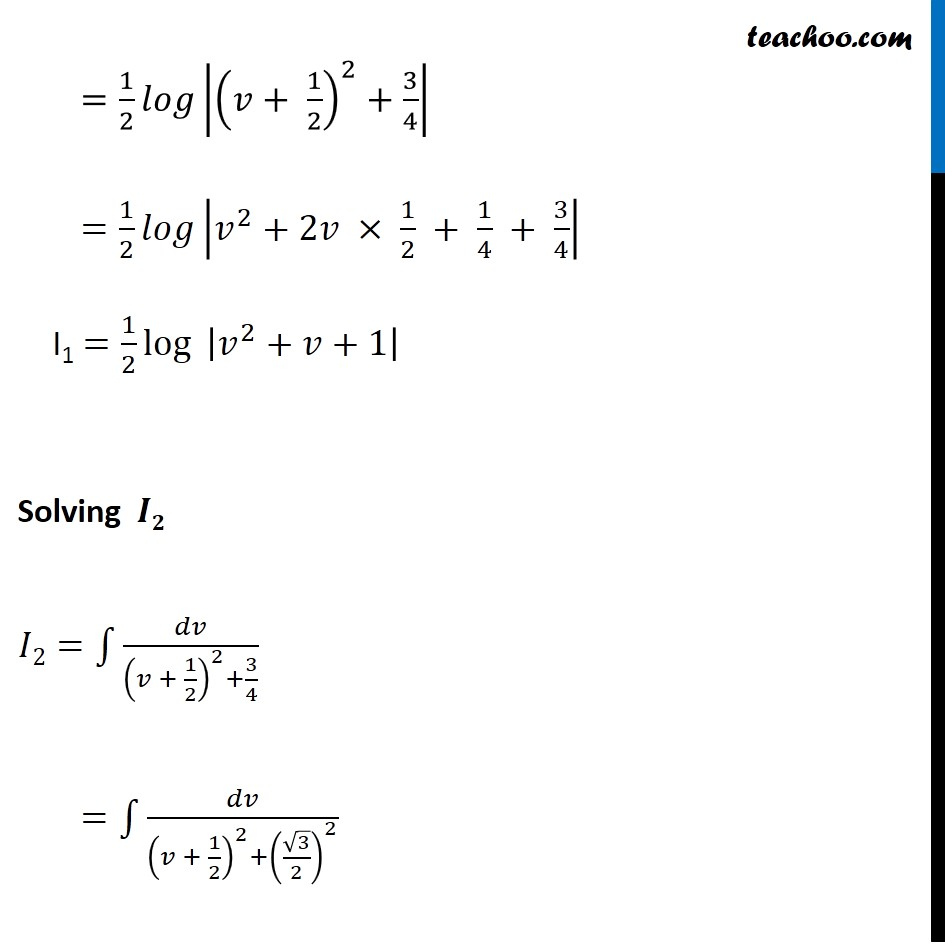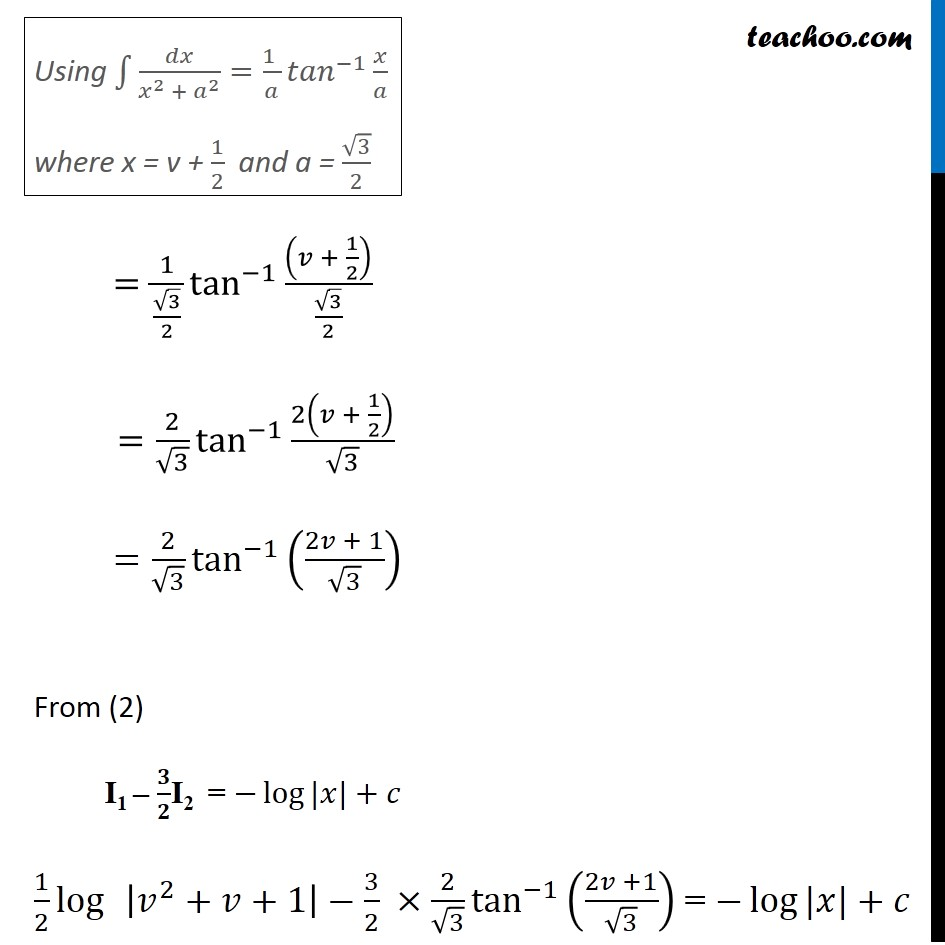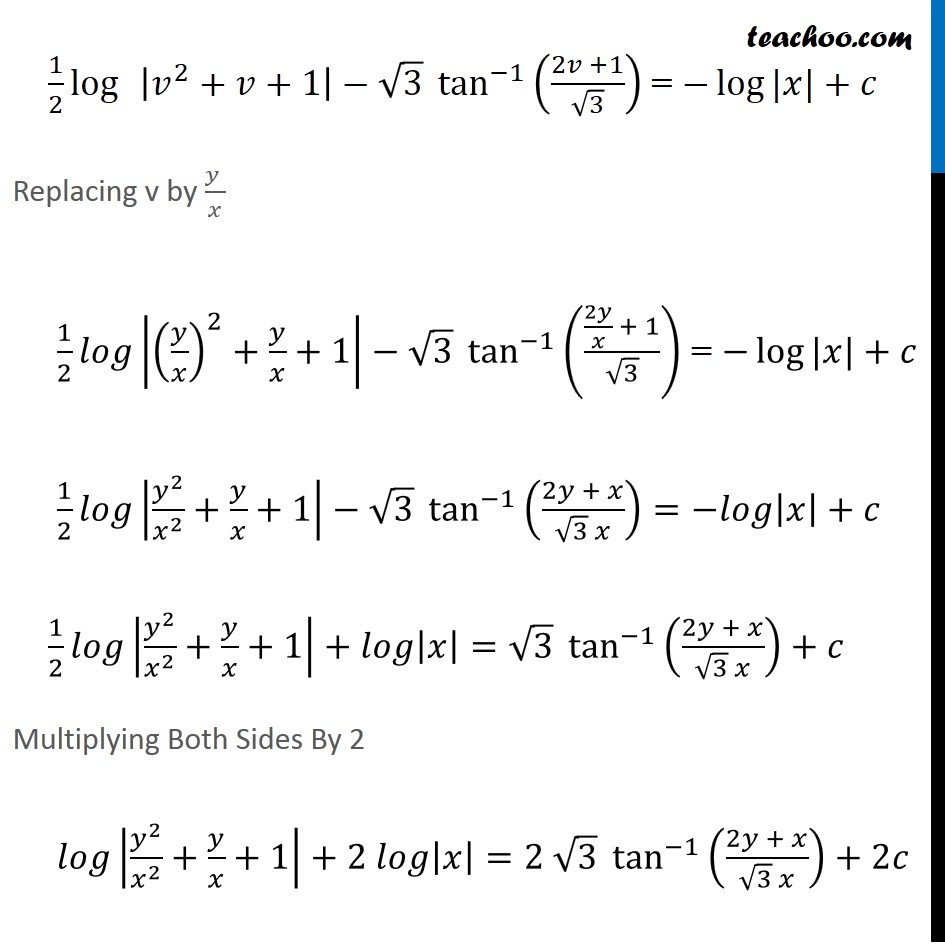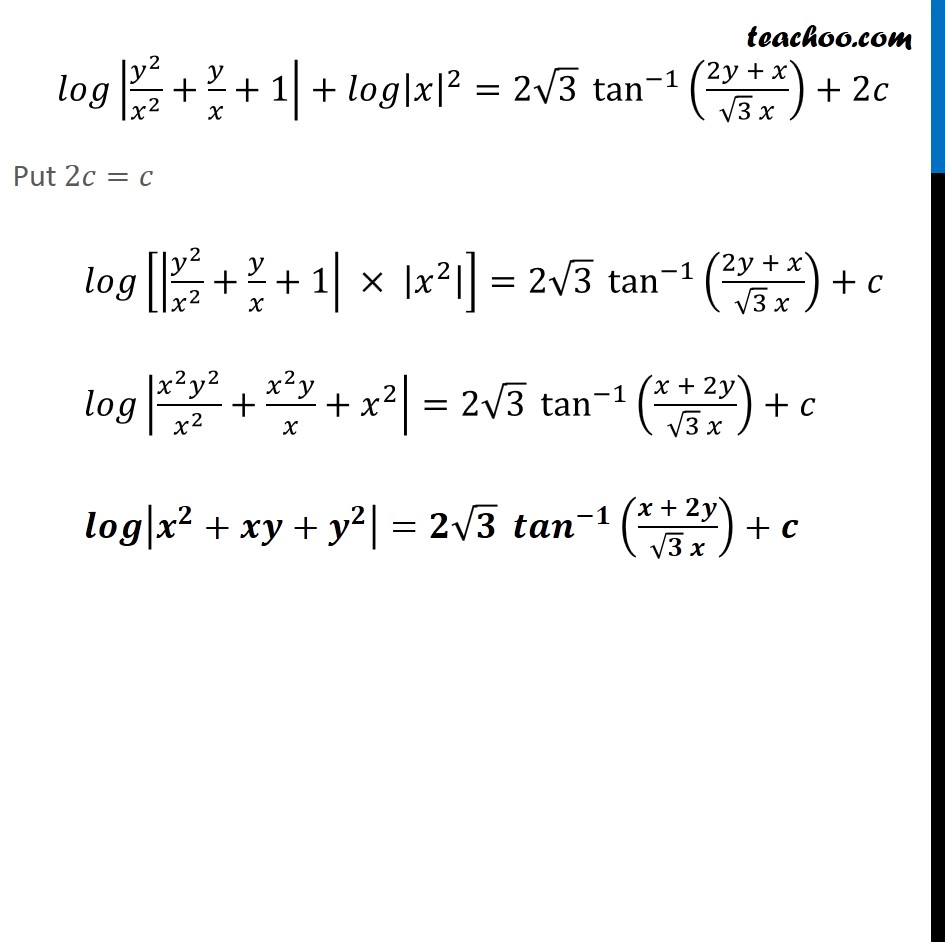1. Chapter 9 Class 12 Differential Equations (Term 2)
2. Serial order wise
3. Examples

Transcript

Example 15 Show that the differential equation (𝑥−𝑦) 𝑑𝑦/𝑑𝑥=𝑥+2𝑦 is homogeneous and solve it. Step 1: Find 𝑑𝑦/𝑑𝑥 (𝑥−𝑦) 𝑑𝑦/𝑑𝑥=𝑥+2𝑦 𝑑𝑦/𝑑𝑥=((𝑥 + 2𝑦)/(𝑥 − 𝑦)) Step 2: Put F(𝑥 , 𝑦)=𝑑𝑦/𝑑𝑥 & Find F(𝜆𝑥 ,𝜆𝑦) 𝑑𝑦/𝑑𝑥=((𝑥 + 2𝑦)/(𝑥 − 𝑦)) Put F(𝑥 , 𝑦)=((𝑥 + 2𝑦)/(𝑥 − 𝑦)) Finding F(𝛌𝐱 ,𝛌𝐲) F(𝜆𝑥 ,𝜆𝑦)=(𝜆𝑥 + 2(𝜆𝑦))/(𝜆𝑥 −𝜆𝑦) =𝜆(𝑥 + 2𝑦)/(𝜆 (𝑥 − 𝑦) ) =((𝑥 + 2𝑦))/(𝑥 − 𝑦) = F(𝑥 , 𝑦) Thus , F(𝜆𝑥 ,𝜆𝑦)="F" (𝑥 , 𝑦)" " =𝜆°" F" (𝑥 , 𝑦)" " Thus , "F" (𝑥 , 𝑦)" is Homogeneous function of degree zero" Therefore, the given Differential Equation is Homogeneous differential Equation Step 3: Solving 𝑑𝑦/𝑑𝑥 by Putting 𝑦=𝑣𝑥 𝑑𝑦/𝑑𝑥=((𝑥 + 2𝑦)/(𝑥 − 𝑦)) Let 𝑦=𝑣𝑥 So , 𝑑𝑦/𝑑𝑥=𝑑(𝑣𝑥)/𝑑𝑥 𝑑𝑦/𝑑𝑥=𝑑𝑣/𝑑𝑥 . 𝑥+𝑣 𝑑𝑥/𝑑𝑥 𝑑𝑦/𝑑𝑥=𝑑𝑣/𝑑𝑥 𝑥+𝑣 Putting 𝑑𝑦/𝑑𝑥 𝑎nd 𝑦/𝑥 𝑖𝑛 (1) 𝑑𝑦/𝑑𝑥=(𝑥 + 2𝑦)/(𝑥 − 𝑦) 𝑑𝑣/𝑑𝑥 𝑥+𝑣= (𝑥 + 2𝑣𝑥)/(𝑥 − 𝑣𝑥) 𝑑𝑣/𝑑𝑥 𝑥+𝑣= 𝑥(1 + 2𝑣)/𝑥(1 − 𝑣) 𝑑𝑣/𝑑𝑥 𝑥+𝑣= (1 + 2𝑣)/(1 − 𝑣) 𝑑𝑣/𝑑𝑥 𝑥= (1 + 2𝑣)/(1 − 𝑣)−𝑣 𝑑𝑣/𝑑𝑥 . 𝑥= (1 + 2𝑣 − 𝑣 (1 − 𝑣))/(1 − 𝑣) 𝑑𝑣/𝑑𝑥 . 𝑥= (1 + 2𝑣 − 𝑣 +〖 𝑣〗^2)/(1 − 𝑣) 𝑑𝑣/𝑑𝑥 . 𝑥= (〖 𝑣〗^2 + 𝑣 + 1)/(1 − 𝑣) 𝑑𝑣/𝑑𝑥 𝑥=−((〖 𝑣〗^2 + 𝑣 + 1)/(𝑣 − 1)) 𝑑𝑣((𝑣 − 1)/(𝑣^(2 )+ 𝑣 + 1))=(−𝑑𝑥)/𝑥 Integrating Both Sides ∫1▒〖(𝑣 − 1)/(𝑣^2 + 𝑣 + 1) 𝑑𝑣=∫1▒(−𝑑𝑥)/𝑥〗 ∫1▒〖(𝑣 − 1)/(𝑣^2 + 𝑣 + 1) 𝑑𝑣=−∫1▒𝑑𝑥/𝑥〗 ∫1▒〖((𝑣 − 1) 𝑑𝑣)/(𝑣^2 + 𝑣 + 1) 𝑑𝑣〗=−log⁡〖|𝑥|〗 + 𝑐 We can write 𝑣^2+𝑣+1 = 𝑣^2 + 1/2 . 2v + (1/2)^2+1−(1/2)^2 =(𝑣+1/2)^2 + 1 – 1/4 =(𝑣+1/2)^2+3/4 Putting 𝑣^2+𝑣+1=(𝑣+1/2)^2+3/4 and 𝑣−1=𝑣+𝟏/𝟐−𝟏/𝟐−1 =(𝑣+1/2)−3/2 ∫1▒((𝑣 + 1/2) − 3/2)/((𝑣 + 1/2)^2+ 3/4) 𝑑𝑣=−log⁡〖|𝑥|+𝑐〗 ∫1▒(𝑣 + 1/2)/((𝑣 + 1/2)^2+ 3/4) 𝑑𝑣−3/2 ∫1▒1/((𝑣 + 1/2)^2+ 3/4) 𝑑𝑣=−log⁡〖|𝑥|+𝑐〗 So, our equation becomes I1 – 𝟑/𝟐I2 = −log⁡〖|𝑥|〗+𝑐 Solving 𝑰𝟏 𝐼1=∫1▒((𝑣 + 1/2))/((𝑣 + 1/2)^2+ 3/4) 𝑑𝑣 Put (𝑣+ 1/2)^2+ 3/4 =𝑡 Diff. w.r.t. 𝑣 𝑑((𝑣 + 1/2)^2+ 3/4)/𝑑𝑣=𝑑𝑡/𝑑𝑣 2(𝑣+1/2)=𝑑𝑡/𝑑𝑣 𝑑𝑣=𝑑𝑡/2(𝑣 + 1/2) Putting value of v & dv in I1 𝐼1=∫1▒((𝑣 + 1/2))/𝑡 ×𝑑𝑡/2(𝑣 + 1/2) =1/2 ∫1▒𝑑𝑡/𝑡 =1/2 log⁡ |𝑡| Putting back 𝑡=(𝑣+ 1/2)^2+3/4 =1/2 𝑙𝑜𝑔|(𝑣+ 1/2)^2+3/4| =1/2 𝑙𝑜𝑔|𝑣^2+2𝑣 × 1/2 + 1/4 + 3/4| I1 =1/2 log⁡〖 |𝑣^2+𝑣+1|〗 Solving 𝑰𝟐 𝐼2=∫1▒𝑑𝑣/((𝑣 + 1/2)^2+3/4) =∫1▒𝑑𝑣/((𝑣 + 1/2)^2+(√3/2)^2 ) Using ∫1▒〖𝑑𝑥/(𝑥^2 + 𝑎^2 )=(1 )/𝑎 〖𝑡𝑎𝑛〗^(−1)⁡〖𝑥/𝑎〗 〗 where x = v + 1/2 and a = √3/2 =1/(√3/2) tan^(−1)⁡〖((𝑣 + 1/2))/(√3/2)〗 =2/√3 tan^(−1)⁡〖2(𝑣 + 1/2)/√3〗 =2/√3 tan^(−1)⁡((2𝑣 + 1)/√3) From (2) I1 – 𝟑/𝟐I2 = −log⁡〖|𝑥|+𝑐〗 1/2 log⁡ |𝑣^2+𝑣+1|−3/2 ×2/√3 tan^(−1)⁡((2𝑣 +1)/√3) = −log⁡〖|𝑥|+𝑐〗 1/2 log⁡ |𝑣^2+𝑣+1|−√3 tan^(−1)⁡((2𝑣 +1)/√3) = −log⁡〖|𝑥|+𝑐〗 Replacing v by (𝑦 )/𝑥 1/2 𝑙𝑜𝑔|(𝑦/𝑥)^2+𝑦/𝑥+1|−√3 tan^(−1)⁡((2𝑦/𝑥 + 1)/√3) = −log⁡〖|𝑥|+𝑐〗 1/2 𝑙𝑜𝑔|𝑦^2/𝑥^2 +𝑦/𝑥+1|−√3 tan^(−1)⁡((2𝑦 + 𝑥)/(√3 𝑥))=−𝑙𝑜𝑔|𝑥|+𝑐 1/2 𝑙𝑜𝑔|𝑦^2/𝑥^2 +𝑦/𝑥+1|+𝑙𝑜𝑔|𝑥|=√3 tan^(−1)⁡((2𝑦 + 𝑥)/(√3 𝑥))+𝑐 Multiplying Both Sides By 2 𝑙𝑜𝑔|𝑦^2/𝑥^2 +𝑦/𝑥+1|+2 𝑙𝑜𝑔|𝑥|=2 √3 tan^(−1)⁡((2𝑦 + 𝑥)/(√3 𝑥))+2𝑐 𝑙𝑜𝑔|𝑦^2/𝑥^2 +𝑦/𝑥+1|+𝑙𝑜𝑔|𝑥|^2=2√3 tan^(−1)⁡((2𝑦 + 𝑥)/(√3 𝑥))+2𝑐 𝑙𝑜𝑔|𝑦^2/𝑥^2 +𝑦/𝑥+1|+𝑙𝑜𝑔|𝑥|^2=2√3 tan^(−1)⁡((2𝑦 + 𝑥)/(√3 𝑥))+2𝑐 Put 2𝑐=𝑐 𝑙𝑜𝑔[|𝑦^2/𝑥^2 +𝑦/𝑥+1| × |𝑥^2 |]=2√3 tan^(−1)⁡((2𝑦 + 𝑥)/(√3 𝑥))+𝑐 𝑙𝑜𝑔|〖𝑥^2 𝑦〗^2/𝑥^2 +(𝑥^2 𝑦)/𝑥+𝑥^2 |=2√3 tan^(−1)⁡((𝑥 + 2𝑦)/(√3 𝑥))+𝑐 𝒍𝒐𝒈|𝒙^𝟐+𝒙𝒚+𝒚^𝟐 |=𝟐√𝟑 〖𝒕𝒂𝒏〗^(−𝟏)⁡((𝒙 + 𝟐𝒚)/(√𝟑 𝒙))+𝒄